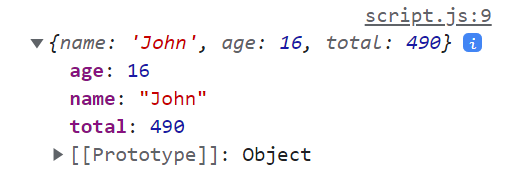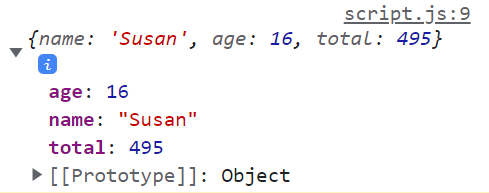# How to search an array of objects in JavaScript?

In this tutorial, let’s look at how to use JavaScript to search an array of objects.

There are multiple ways to search for the properties inside of an object. For example, you can match one of the property values and extract the object that contains that value. Or you can just determine if that particular property value is present in one of the objects in the array and return true if that is the case.

Let’s look at them all in this tutorial.

## Looping through an array

Looping is a very common way of doing this. Let’s say we have an array “students”, and within it, we have data on each student in the form of an object.

Now, you can just use a for loop to loop through this array. Start the loop from 0 because array indices start from 0 but stop right before the length of the array.

So, we’ll loop through 0 to array.length-1.

For every iteration of the loop, let’s check whether the “name” property of the object in that particular iteration is equal to “John” (our condition).

If that’s the case, let’s print out that object.

```let students = [
{name: 'John', age: 16, total: 490},
{name: 'Amber', age: 15, total: 450},
{name: 'Susan', age: 16, total: 495}
];

for(let i=0; i<students.length; i++) {
if(students[i].name == 'John') {
console.log(students[i]);
}
}
```

Run the above lines of code, and you’ll get:As you can see, we extracted “John’s” details, as per our condition.

You can use this method to find any value inside an object, or even print multiple objects that confirm to the given condition. For example, if we were looking to extract the details of students who’ve scored a total of 450 and more, we’ll get 2 results, instead of 1.

## Using forEach to search an array of objects in JavaScript – shorter method

Instead of using a for loop, we can use the forEach loop and achieve the same result with fewer lines of code. The forEach loop is used to loop through every element in an array.

In our piece of code, we’re calling the forEach method on our “students” array. It takes one argument, where you can tell the browser what needs to be done for each iteration.

We’ve created a temporary argument “student” for our arrow function. This argument will contain an element of the array in the order it was placed in.

For this example, why don’t we ask for “Susan’s” details to be printed instead?

```students.forEach(student => {
if(student.name == 'Susan') {
console.log(student);
}
})
```

When we run the above lines of code, we’ll get:## Using the find method to search an array of objects

Though the forEach method simplifies things a bit, we’re still using too many lines of code. By using the “find” method, we can finish everything in just one line of code.

This is a pre-defined JavaScript method for arrays, and it can be used to return the first occurrence of the element that confirms to the given condition. Let’s use the same array of objects example as above, but now, we’re going to attach the “find” method to that array.

For every iteration, the method creates a temporary “student” variable that contains an element of the array (in this case, the student object) in order. Instead of using an “if” statement, we can just give the condition we need checked for every iteration.

The “find” method does not affect the original “students” array, but just extracts the first element that matches the condition. So, we need to assign the extracted value to a variable in order to access it, as shown below:

```let obj = students.find(student => student.name == 'John');
console.log(obj);
```

If you run the above line of code, you’ll get the same result as the first example; that is, the program will extract “John’s” student details.

## Using the findIndex method to find the index of the matched object

Instead of using the find” method, we can also use the findIndex method. This returns the index of the first element that matches the given condition, which can later be used to access the element, if needed.

For this example, let’s use findIndex to retrieve the first object whose “name” property is equal to “Susan”.

```let index = students.findIndex(student => student.name == 'Susan');
console.log(students[index]);
```

When you run the above lines of code, your program will access and retrieve “Susan’s” student details, as needed.

## Using the filter method to filter out all the matching objects

Filter is a pre-defined JavaScript method for arrays that can be used to filter out and return all the objects that pass the given condition.

It follows the same syntax as the “find” and “findIndex” methods.

In this example, let’s use the filter method to extract the details of all students whose age is 16.

```let result = students.filter(student => student.age == 16);
console.log(result);
```

When we run the above lines of code, we’ll end up with an array of 2 objects in our “results” variable, as shown below:We can print out the entire array or manipulate it in any other way. Instead, we can use foreach to print the property values of the objects in the resultant array.

So, that’s it! These are the different ways to search array of objects in JavaScript.